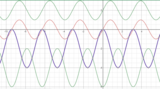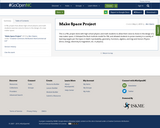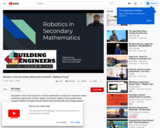Updating search results...

# 5 Results

View
Selected filters:
• TrigonometryUnrestricted Use
CC BY
Rating
0.0 stars

This is an exploration activity for students to visually see the graph of trig functions and understand about sin and cos functions and understand the concept of amplitude.

Subject:
Math 3
Mathematics
Pre-Calculus
Trigonometry
Material Type:
Activity/Lab
Author:
VIJAYALAKSHMI SANKARAN
05/27/2020Unrestricted Use
CC BY
Rating
0.0 stars

This is an exploration activity for students to visually see the graph of trig functions and understand about sin and cos functions and understand the concept of amplitude.Section 2 of this activity allows students to explore the period and vertical shift in trig functions.

Subject:
Math 3
Mathematics
Pre-Calculus
Trigonometry
Material Type:
Activity/Lab
Author:
VIJAYALAKSHMI SANKARAN
05/28/2020Conditional Remix & Share Permitted
CC BY-NC
Rating
0.0 stars

A PBL project that allows high school physics and math students exercise voice & choice in the design of a new maker space.

Subject:
Algebra
Applied Science
Engineering
Geometry
Math 2
Mathematics
Physics
Science
Trigonometry
Material Type:
Unit of Study
11/06/2019Unrestricted Use
CC BY
Rating
0.0 stars

This is an exploration activity for students to visually see the graph of trig functions and understand about sin and cos functions and understand the concept of amplitude.and periods. Thanks to the original author, VIJAYLAKSHMI SANKARAN for sharing and allowing remix access to this resource.

Subject:
Math 3
Mathematics
Pre-Calculus
Trigonometry
Material Type:
Activity/Lab
Self Assessment
Simulation
Author:
Sheree Osbourne-Dixon
06/01/2020Conditional Remix & Share Permitted
CC BY-NC-SA
Rating
0.0 stars

Using Sphero robots in the classroom to enforce mathematical concepts and connections related to geometry, trigonometry, and basic algebra and introduce students to basic programming concepts. Students can explore through different tasks that deal with rate of change, distance formula (Pythagorean Theorem), angle of rotation (basic trigonometry), and related topics while applying those concepts to a physical activity. The Sphero tools also allow students with different programming experiences to tailor their work to fit their skill level from basic (block programming) to advanced (Java). The tasks also promote collaboration between students to problem solve issues on the fly as they work to reach a common goal or compete to outdo other groups.

https://www.desmos.com/calculator/dld4f1cbgm

Subject:
Algebra
Geometry
Mathematics
Professional Development
STEM
Science
Trigonometry
Material Type:
Teaching/Learning Strategy